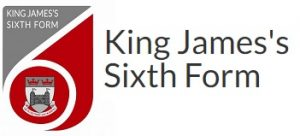## Mathematics - Further

Further Mathematics is appropriate for students with an enthusiasm for mathematics. It prepares students for further study and employment in highly mathematical disciplines that require knowledge and understanding of sophisticated mathematical ideas and techniques.

The course will suit the able student who enjoys mathematics and wishes to understand it in more depth. Desirable qualities include initiative and independence of learning as the subjects covered are diverse.

Pure Mathematics represents two thirds of the content with the other third being split between the Discrete and Statistics branches of mathematics.

The Pure Mathematics content not only extends some of the topics from the Mathematics A Level but also provides the opportunity to study different topics such as complex numbers, matrices, polar coordinates, hyperbolic functions and differential equations.

The Statistics elements build on the content covered in the Mathematics A Level whilst the Discrete Mathematics element introduces the use and analysis of algorithms. The topics covered include graphs and networks, linear programming and critical path analysis.

## To get started

Students studying Further Mathematics must also be studying A Level Mathematics and should have achieved at least grade 7 at GCSE.

## Exam and Assessment Information

AQA – 7367

As well as sitting the A Level Mathematics examinations, students will sit three extra examinations each lasting two hours.

Paper 1 : Pure Mathematics

Paper 2 : Pure Mathematics

Paper 3 : Statistics and Discrete Maths

An advanced scientific calculator is required for all examinations.

## What Next?

Further Mathematics is ideal for any student wishing to study a degree course with a high mathematical content such as mathematics, engineering disciplines, the sciences and economics. Many students comment that the first year of their Mathematics degree is made much easier with this qualification and in many ways represents revision of the work covered in Further Mathematics.

## PLEASE SELECT FROM THE OPTIONS BELOWThe absence reporting form is currently under review and will be available from Friday 22nd April.

We apologise for any inconvenience